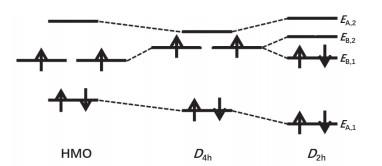## A Modified Hückel Molecular Orbital Model to Predict the Structure of Cyclo[2n]carbons

Zhang Yichi, Hou Hua, Wang Baoshan,

 基金资助: 武汉大学通识课程教学改革项目Abstract

Hückel Molecular Orbital (HMO) theory is popular in chemistry, but it assumes that the reduced resonance integrals exist only between the nearest-neighbor carbon atoms. Such an approximation has been justified by the explicit inclusion of the resonance integrals between the meta-directing π-bonded atoms. The energy eigenvalues are obtained using the symmetry-adapted molecular orbitals, together with the extra stabilization energy due to symmetry breaking. The polyynic geometries of cyclo[2n]carbons with alternating angles are explained rationally using the modified HMO model, which gains new insights on the second-order Jahn-Teller effect for carbon rings.

Keywords： Hückel molecular orbital theory ; Resonance integral ; Carbon ring ; Symmetry ; Energy level

Zhang Yichi. A Modified Hückel Molecular Orbital Model to Predict the Structure of Cyclo[2n]carbons. University Chemistry[J], 2022, 37(1): 2104033-0 doi:10.3866/PKU.DXHX202104033

### 图1C2n分子结构对称性降低现象可以用二级Jahn-Teller效应结合高级电子相关计算(例如Diffusion Monte Carlo)加以解释，但该方法过于深奥，难以在本科生教学课堂中加以推广。更重要的是，针对纯碳环分子结构的计算结果严重依赖于理论方法，不同理论水平甚至得到截然相反的结论。例如流行的密度泛函理论(DFT)方法预测C18的cumulenic结构更稳定，而耦合蔟(CCSD)方法则支持C18的polyynic结构更稳定。因此，需要一种新的简便模型或理论能够正确解释C2n分子结构难题。

HMO理论假设不相邻原子之间的共振积分为零。通常情况下此近似是合理的，因为不相邻原子之间的距离远大于相邻原子的距离。但是，从图 1的C6分子结构可以看出：当对称性从D6h降为D3h时，处于间位碳原子之间的距离(1, 3)、(1, 5)、(3, 5)将明显缩短，这些碳原子之间的共振积分显然不宜忽略，其可能是导致C2n分子通过降低对称性而获得额外稳定化能的直接原因。因此，本文针对HMO模型进行了改进，明确考虑间位碳原子之间的共振积分，成功解释了C2n分子的结构特征。

### 1.1 C4分子

C4分子cumulenic与polyynic结构的点群分别为D4h (正方形)与D2h (菱形)，如图 2所示。HMO模型仅需考虑点群绕主轴的旋转操作，D4hD2h对称性约束下的分子轨道均可按照C2点群特征标表的不可约表示进行分类：pʹ1变换至pʹ1pʹ3pʹ2变换至pʹ2pʹ4，因此基轨道可取为(pʹ1, pʹ2)，其不可约表示为：

${\mathit{\Gamma }_{\left( {p_1^{'}, p_2^{'}} \right)}} = A \oplus B$

### 图2pʹ1pʹ2分别应用不可约表示AB的投影算符，得到归一化对称性匹配的分子轨道：

${{ψ}}_{{A}{, 1}}{}{=}{}\frac{{1}}{\sqrt{{2}}}\left({{p}}_{{1}}^{{'}}{+}{}{{p}}_{{3}}^{{'}}\right){}\;\;{{ψ}}_{{A}{, 2}}{}{=}{}\frac{{1}}{\sqrt{{2}}}\left({{p}}_{{2}}^{{'}}{}{+}{}{{p}}_{{4}}^{{'}}\right)$

${{ψ}}_{{B}{, 1}}{}{=}{}\frac{{1}}{\sqrt{{2}}}\left({{p}}_{{1}}^{{'}}{-}{}{{p}}_{{3}}^{{'}}\right){}\;\;{{ψ}}_{{B}{, 2}}{}{=}{}\frac{{1}}{\sqrt{{2}}}\left({{p}}_{{2}}^{{'}}{}{-}{}{{p}}_{{4}}^{{'}}\right)$

AB两种不可约表示的分子轨道分别计算轨道积分：

${{H}}_{{A}{, 1;}{A}{, 1}}{=}\frac{{1}}{{2}}\left({{p}}_{{1}}^{{'}}|\widehat{{H}}|{{p}}_{{1}}^{{'}}{}{+}{}{2}{{p}}_{{1}}^{{'}}|\widehat{{H}}|{{p}}_{{3}}^{{'}}{}{+}{}{{p}}_{{3}}^{{'}}|\widehat{{H}}|{{p}}_{{3}}^{{'}}\right)$

${{H}}_{{A}{, 1;}{A}{, 1}}{=}\frac{{1}}{{2}}\left({{p}}_{{1}}^{{'}}|\widehat{{H}}|{{p}}_{{2}}^{{'}}{}{+}{}{{p}}_{{2}}^{{'}}|\widehat{{H}}|{{p}}_{{3}}^{{'}}{}{+}{}{{p}}_{{3}}^{{'}}|\widehat{{H}}|{{p}}_{{4}}^{{'}}{}{+}{}{{p}}_{{4}}^{{'}}|\widehat{{H}}|{{p}}_{{1}}^{{'}}\right)$

${{H}}_{{A}{, 2;}{A}{, 2}}{}{=}{}\frac{{1}}{{2}}\left({{p}}_{{2}}^{{'}}|\widehat{{H}}|{{p}}_{{2}}^{{'}}{}{+}{}{2}{{p}}_{{2}}^{{'}}|\widehat{{H}}|{{p}}_{{4}}^{{'}}{}{+}{}{{p}}_{{4}}^{{'}}|\widehat{{H}}|{{p}}_{{4}}^{{'}}\right)$

${{H}}_{{B}{, 1;}{B}{, 1}}{}{=}{}\frac{{1}}{{2}}\left({{p}}_{{1}}^{{'}}|\widehat{{H}}|{{p}}_{{1}}^{{'}}{}{-}{}{2}{{p}}_{{1}}^{{'}}|\widehat{{H}}|{{p}}_{{3}}^{{'}}{}{+}{}{{p}}_{{3}}^{{'}}|\widehat{{H}}|{{p}}_{{3}}^{{'}}\right)$

${{H}}_{{B}{, 1;}{B}{, 2}}{}{=}{}\frac{{1}}{{2}}\left({{p}}_{{1}}^{{'}}|\widehat{{H}}|{{p}}_{{2}}^{{'}}{-}{{p}}_{{2}}^{{'}}|\widehat{{H}}|{{p}}_{{3}}^{{'}}{}{+}{}{{p}}_{{3}}^{{'}}|\widehat{{H}}|{{p}}_{{4}}^{{'}}{}{-}{}{{p}}_{{4}}^{{'}}|\widehat{{H}}|{{p}}_{{1}}^{{'}}\right)$

${{H}}_{{B}{, 2;}{B}{, 2}}{}{=}{}\frac{{1}}{{2}}\left({{p}}_{{2}}^{{'}}|\widehat{{H}}|{{p}}_{{2}}^{{'}}{}{-}{}{2}{{p}}_{{2}}^{{'}}|\widehat{{H}}|{{p}}_{{4}}^{{'}}{}{+}{}{{p}}_{{4}}^{{'}}|\widehat{{H}}|{{p}}_{{4}}^{{'}}\right)$

${{p}}_{{1}}^{{'}}|\widehat{{H}}|{{p}}_{{1}}^{{'}}{}{=}{}{{p}}_{{2}}^{{'}}|\widehat{{H}}|{{p}}_{{2}}^{{'}}{}{=}{}{{p}}_{{3}}^{{'}}|\widehat{{H}}|{{p}}_{{3}}^{{'}}{}{=}{}{{p}}_{{4}}^{{'}}|\widehat{{H}}|{{p}}_{{4}}^{{'}}{}{=}{}{α}$

${{p}}_{{1}}^{{'}}|\widehat{{H}}|{{p}}_{{2}}^{{'}}{}{=}{}{{p}}_{{2}}^{{'}}|\widehat{{H}}|{{p}}_{{3}}^{{'}}{}{=}{}{{p}}_{{3}}^{{'}}|\widehat{{H}}|{{p}}_{{4}}^{{'}}{}{=}{}{{p}}_{{4}}^{{'}}|\widehat{{H}}|{{p}}_{{1}}^{{'}}{}{=}{}{β}$

${{p}}_{{1}}^{{'}}|\widehat{{H}}|{{p}}_{{3}}^{{'}}{}{=}{}{{β}}_{\rm{short}}$

${{p}}_{{2}}^{{'}}|\widehat{{H}}|{{p}}_{{4}}^{{'}}{=}{{β}}_{\rm{long}}$

${{H}}_{{A}{, 1;}{A}{, 1}}{}{=}{}{α}{}{+}{}{{β}}_{\rm{short}}\;\;{{H}}_{{A}{, 1;}{A}{, 2}}{}{=}{}{2}{β}\;\;{{H}}_{{A}{, 2;}{A}{, 2}}{}{=}{}{α}{}{+}{}{{β}}_{\rm{long}}$

${{H}}_{{B}{, 1;}{B}{, 1}}{}{=}{}{α}{}{-}{}{{β}}_{\rm{short}}\;\;{{H}}_{{B}{, 1;}{B}{, 2}}{}{=}{}{0}\;\;{{H}}_{{B}{, 2;}{B}{, 2}}{}{=}{}{α}{{}{-}{}{β}}_{\rm{long}}$

$\left|\begin{array}{l}{{H}}_{{A}{, 1;}{A}{, 1}}{}{-}{}{E}& {{H}}_{{A}{, 1;}{A}{, 2}}\\ {{H}}_{{A}{, 1;}{A}{, 2}}& {{H}}_{{A}{, 2;}{A}{, 2}{}}{-}{}{E}\end{array}\right|{=}{}{0}$

$\left|\begin{array}{l}{{H}}_{{B}{, 1;}{B}{, 1}}{}{-}{}{E}& {{H}}_{{B}{, 1;}{B}{, 2}}\\ {{H}}_{{B}{, 1;}{B}{, 2}}& {{H}}_{{B}{, 2;}{B}{, 2}{}}{-}{}{E}\end{array}\right|{=}{}{0}$

${{E}}_{{A}}{}{=}{}{α}{}{+}{}\frac{{{β}}_{\rm{long}}{}{+}{}{{β}}_{\rm{short}}}{{2}}{±}\frac{\sqrt{{\left({{β}}_{\rm{long}}{}{-}{}{{β}}_{\rm{short}}\right)}^{{2}}{}{+}{}{16}{{β}}^{{2}}}}{{2}}$

${{E}}_{{B}}{}{=}{}{α}{}{-}{}\frac{{{β}}_{\rm{long}}{}{+}{}{{β}}_{\rm{short}}}{{2}}{}{±}{}\frac{{{β}}_{\rm{long}}{}{-}{{}{β}}_{\rm{short}}}{{2}}$

${{E}}_{{A}}{}{=}{}\frac{{{β}}_{\rm{long}}{}{+}{}{{β}}_{\rm{short}}}{{2}}{±}\frac{\sqrt{{\left({{β}}_{\rm{long}}{}{-}{}{{β}}_{\rm{short}}\right)}^{{2}}{}{+}{}{16}{{β}}^{{2}}}}{{2}}$

${{E}}_{{B}}{}{=}{}{}{-}{}\frac{{{β}}_{\rm{long}}{}{+}{}{{β}}_{\rm{short}}}{{2}}{}{±}{}\frac{{{β}}_{\rm{long}}{}{-}{{}{β}}_{\rm{short}}}{{2}}$

1) 若令βlong = βshort = 0，即不考虑间位原子轨道共振积分的贡献，则(8a–b)式简化为原始HMO的能级公式，即：

${{E}}_{{A}{, {\rm{original}}}}{}{=}{}{±2}{β}$

${{E}}_{{B}{, {\rm{original}}}}{}{=}{}{0}$

2) 对于D4h对称结构(即图 2中正方形)，因对角线相等，βshort = βlong，与之相应的分子轨道的能级分别为：

${{E}}_{{A}{, {\rm{square}}}}{=}\frac{{{β}}_{\rm{long}}{+}{{β}}_{\rm{short}}}{{2}}{}{±}{}{2}{{β}}^{}$

${{E}}_{{B}{, {\rm{square}}}}{=}\frac{{{β}}_{\rm{long}}{+}{{β}}_{\rm{short}}}{{2}}$

### 图33) 对于D2h对称结构(即图 2中菱形)，短对角的两对pʹ原子轨道的相互作用比长对角强，${}\left|{{β}}_{\rm{short}}\right|{}{ > }\left|{{β}}_{\rm{long}}\right|$，即${{β}}_{\rm{short}}{}{ < }{}{{β}}_{\rm{long}}$，相应的分子轨道能级为：

${{E}}_{{A}{, {\rm{rhombus}}}}{}{=}{}\frac{{{β}}_{\rm{long}}{}{+}{}{{β}}_{\rm{short}}}{{2}}{±}\frac{\sqrt{{\left({{β}}_{\rm{long}}{}{-}{}{{β}}_{\rm{short}}\right)}^{{2}}{}{+}{}{16}{{β}}^{{2}}}}{{2}}$

${{E}}_{{B}{, {\rm{rhombus}}}}{=}{-}{}\frac{{{β}}_{\rm{long}}{+}{{β}}_{\rm{short}}}{{2}}{±}\frac{{{β}}_{\rm{long}}{-}{{β}}_{\rm{short}}}{{2}}=\left\{\begin{array}{l}{-}{{β}}_{\rm{short}}\;\;\;{\rm{antibonding}}\\ {-}{{β}}_{\rm{long}}\;\;\;\;\;{\rm{bonding}}\end{array}\right.$

${{E}}_{\rm{rhombus}}{}{=}{}{-}{{β}}_{\rm{long}}{}{+}{}{{β}}_{\rm{short}}{}{-}{}\sqrt{{\left({{β}}_{\rm{long}}{-}{{β}}_{\rm{short}}\right)}^{{2}}{}{+}{}{16}{{β}}^{{2}}}$

D4h变为D2h因对称性降低而获得的额外稳定化能为：

${Δ}{E}{}{=}{}{{E}}_{\rm{square}}{}{-}{}{{E}}_{\rm{rhombus}}{}{=}{}{4}{β}{}{-}\left({-}{{β}}_{\rm{long}}{}{+}{}{{β}}_{\rm{short}}{}{-}{}\sqrt{{\left({{β}}_{\rm{long}}{-}{{β}}_{\rm{short}}\right)}^{{2}}{}{+}{}{16}{{β}}^{{2}}}\right)$

${Δ}{E}{}{=}{}{(}{{β}}_{\rm{long}}{}{-}{}{{β}}_{\rm{short}}{}{)}{}{+}\left({4}{β}{}{+}{}\sqrt{{\left({{β}}_{\rm{long}}{}{-}{{}{β}}_{\rm{short}}\right)}^{{2}}{}{+}{}{16}{{β}}^{{2}}}\right)$

### 1.2 C6分子

${{E}}_{{A}}{=}\frac{\left({{β}}_{\rm{long}}{+}{{β}}_{\rm{short}}\right){±}{}\sqrt{{\left({{β}}_{\rm{long}}{}{-}{}{{β}}_{\rm{short}}\right)}^{{2}}{+ 16}{{β}}^{{2}}}}{{2}}$

${{E}}_{{E}}{=}\frac{{-}\left({{β}}_{\rm{long}}{+}{{β}}_{\rm{short}}\right){±}{}\sqrt{{\left({{β}}_{\rm{long}}{}{-}{}{{β}}_{\rm{short}}\right)}^{{2}}{+ 16}{{β}}^{{2}}}}{{4}}$

${Δ}{E}{}{=}{}{2}{}{×}{}\frac{{-}{4}{β}{}{-}{}\sqrt{{\left({{β}}_{\rm{long}}{}{-}{{}{β}}_{\rm{short}}\right)}^{{2}}{+}{}{16}{{β}}^{{2}}}}{{2}}{}{+}{}{4}{}{×}{}\frac{{-}{4}{β}{}{-}\sqrt{{\left({{β}}_{\rm{long}}{}{-}{}{{β}}_{\rm{short}}\right)}^{{2}}{}{+}{}{16}{{β}}^{{2}}}}{{4}}$

### 图4${Δ}{E}{}{=}{}{2}\left({-}{4}{β}{}{-}{}\sqrt{{\left({{β}}_{\rm{long}}{}{-}{}{{β}}_{\rm{short}}\right)}^{{2}}{}{+}{}{16}{{β}}^{{2}}}\right)$

## 参考文献 原文顺序 文献年度倒序 文中引用次数倒序 被引期刊影响因子

Kutzelnigg W. J. Comput. Chem. 2007, 28 (1), 25.

Hirsch A. Nat. Mater. 2010, 9 (11), 868.

Diederich F. ; Kivala M. Adv. Mater. 2010, 22 (7), 803.

Hutter J. ; Lüthi H. P. ; Diederich F. J. Am. Chem. Soc. 1994, 101 (3), 750.

Martin J. M. L. ; El-Yazal J. ; François J. -P. Chem. Phys. Lett. 1995, 242 (6), 570.

Fowler P. W. ; Mizoguchi N. ; Bean D. E. ; Havenith R. W. A. Chem. Eur. J. 2009, 15 (28), 6964.

Kaiser K. ; Scriven L. M... ; Schulz F. ; Gawel P. ; Gross L. ; Anderson H. L. Science 2019, 365 (6459), 1299.

Arulmozhiraja S. ; Ohno T. J. Chem. Phys. 2008, 128 (11), 114301.

Hong I. ; Ahn J. ; Shin H. ; Bae H. ; Lee H. ; Benali A. ; Kwon Y. J. Phys. Chem. A 2020, 124 (18), 3636.

Liu Z. ; Lu T. ; Chen Q. Carbon 2020, 165, 468.

Pariser R. ; Parr R. G. J. Chem. Phys. 1953, 21 (3), 466.

Hoffmann R. J. Chem. Phys. 1963, 39 (6), 1397.

Wheland G. H. J. Am. Chem. Soc. 1941, 63 (7), 2025.

Wheland G. H. ; Mann D. E. J. Chem. Phys. 1949, 17 (3), 264.

Watts J. D. ; Gauss J. ; Stanton J. F. ; Bartlett R.J. J. Chem. Phys. 1992, 97 (11), 8372.

/

 〈〉